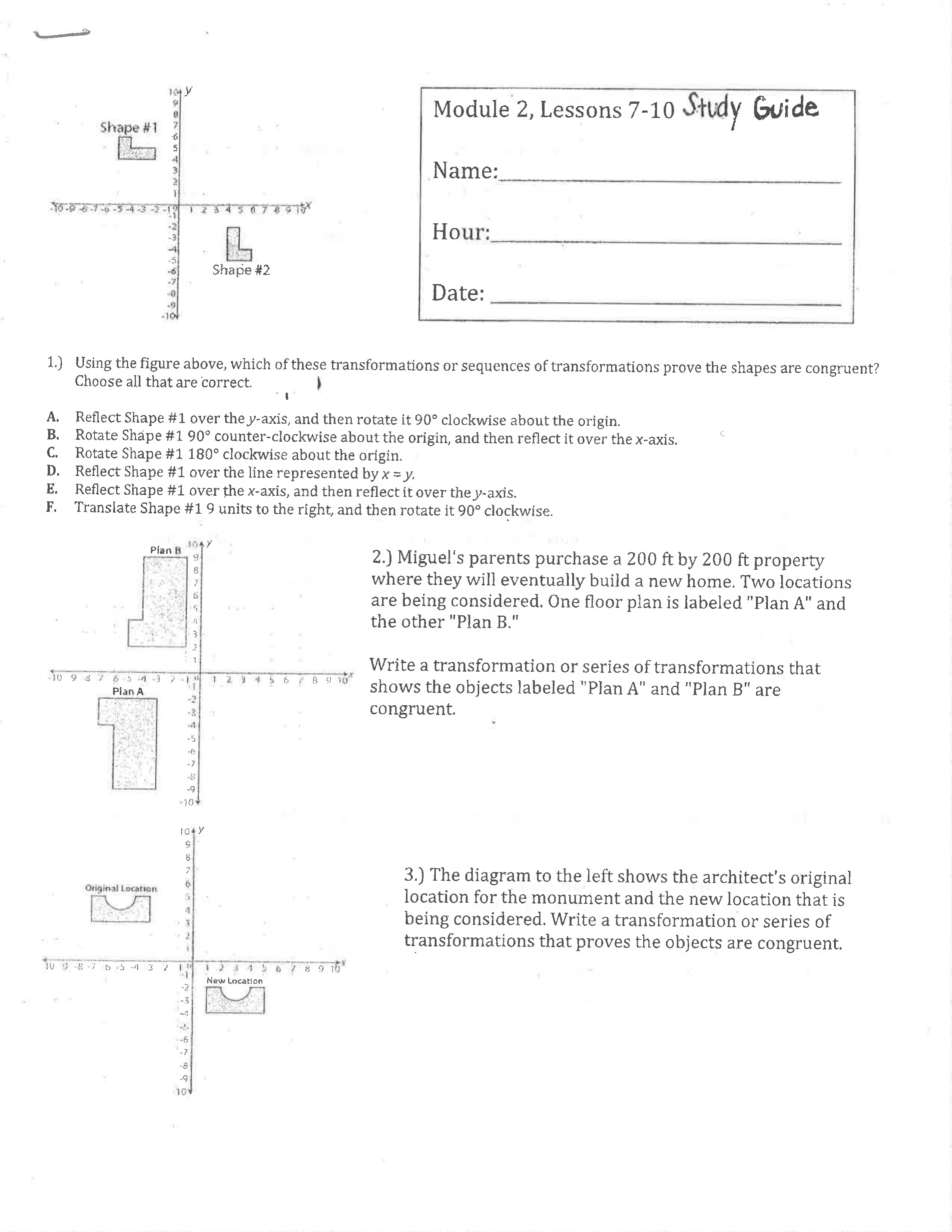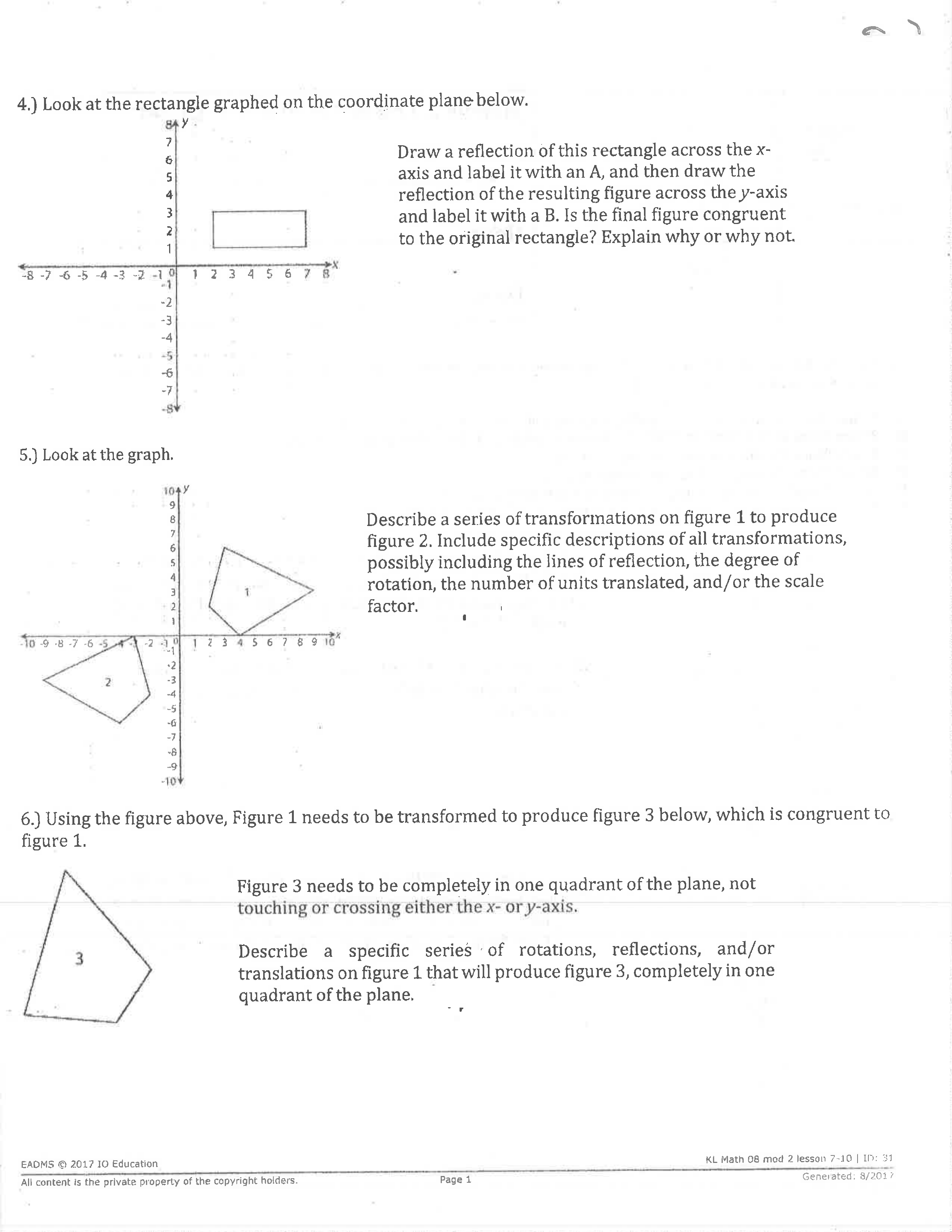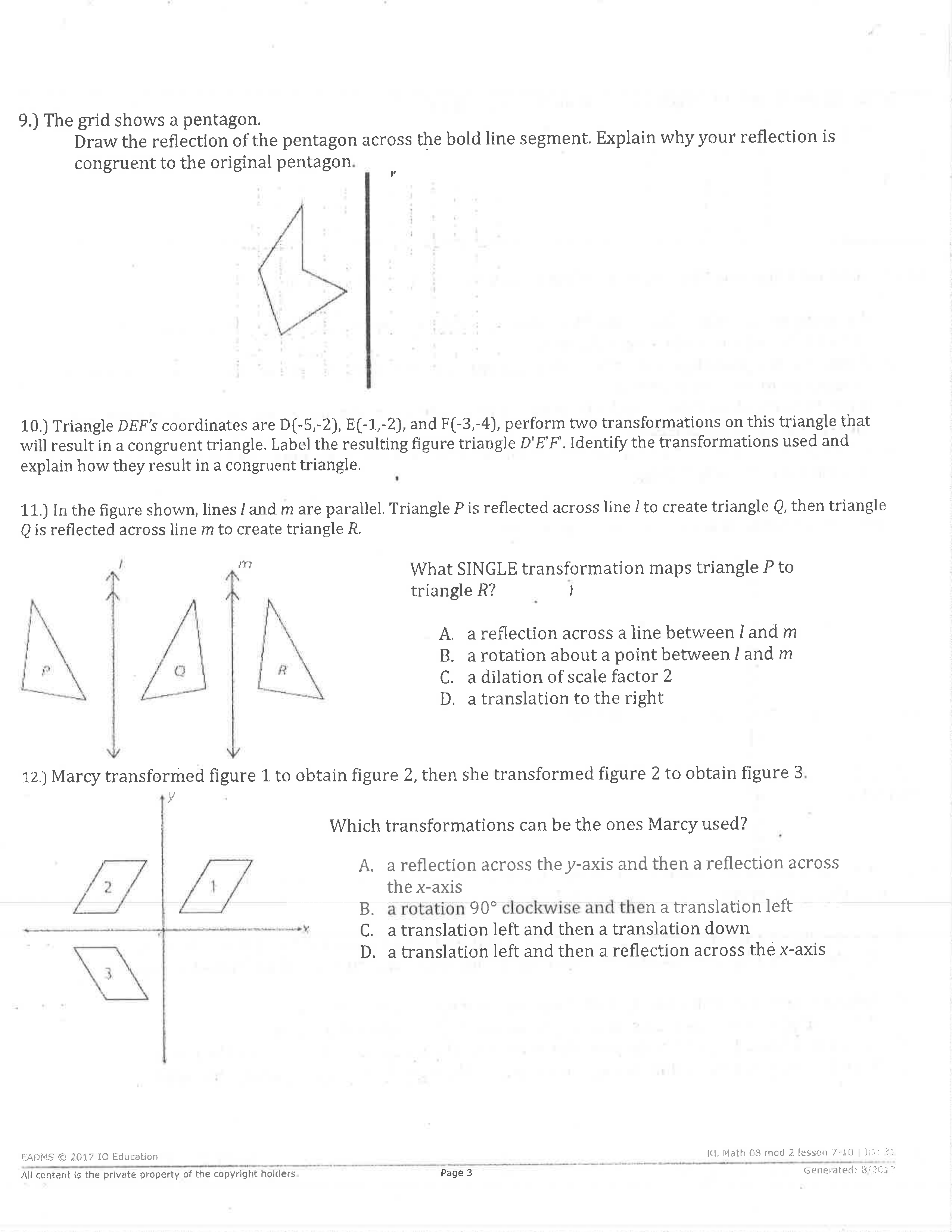Module 2 Topic B Review
starstarstarstarstarstarstarstarstarstar
by KILEY LEONARD
| 20 Questions
Note from the author:
Sequences of Transformations1
2
3
1
1
A
B
C
D
E
F
2
1
Write your sequence of transformation here. Don't forget to say what you're moving, how you're moving it, and along what will you be moving your figure.

3
1
Write your sequence of transformation here. Don't forget to say what you're moving, how you're moving it, and along what will you be moving your figure.4
5
6
4
1
Draw the sequence. Is the image congruent.
Yes, the image is congruent
No, the image is similar
They are neither similar nor congruent
5
1
What sequence of transformations would map Figure 1 onto Figure 2.

6
17
8
7
1
A
B
C
D
8
1
A
B
C
D
E
F9
10
11
12
9
1

10
1
11
1
A
B
C
D
12
1
A
B
C
D13
14
15
13
1

14
1
A
B
C
D
15
1
A
B
C
D
16
1
Lyle graphs triangle ABC on coordinate axes. He performs two transformations on this figure that would result in the congruent triangle XYZ. Which series of transformations did Lyle most likely use?
A translation left and then a dilation with a scale factor of 3
A dilation with a scale factor of 2.5 and then a rotaion 180 degrees counterclockwise.
A rotation 90 degrees clockwise and then a reflection across the y-axis.
A reflection across the x-axis and then a dilation with a scale factor of 0.25
17
1
Megan draws a triangle on a coordinate axes. She reflects the triangle across the y-axis and then translates it 5 units to the right. Which statement is true about the triangle formed from these transformations?
It will be larger than the original triangle
It will be congruent to the original triangle
It will be smaller than the original triangle.
It will be a different shape than the original triangle.
18
1
Point W in quadrant II is reflected across the y-axis and then rotated 90 degrees counterclockwise about the origin. In which quadrant is the image of point W?